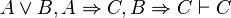# Dilemma facts for kids

Kids Encyclopedia Facts

A dilemma (Greek δί-λημμα "double proposition") is a problem with at least two solutions or possibilities. None of the solutions are practically acceptable; a person in this position has been traditionally described as being impaled on the horns of a dilemma, neither horn being comfortable.

The dilemma is sometimes used as a rhetorical device, in the form "you must accept either A, or B"; here A and B would be propositions each leading to some further conclusion. Applied in this way, it may be a fallacy, a false dichotomy.

In formal logic, the definition of a dilemma differs markedly from everyday usage. Two options are still present, but choosing between them is immaterial because they both imply the same conclusion. Symbolically expressed thus:$A \vee B, A \Rightarrow C, B \Rightarrow C \vdash C$

This can be translated informally as "one (or both) of A or B is known to be true, but they both imply C, so regardless of the truth values of A and B we can conclude C."

Horned dilemmas can present more than two choices. The number of choices of Horned dilemmas can be used in their alternative names, such as two-pronged (two-horned) or dilemma proper, or three-pronged (three-horned) or trilemma, and so on.

Constructive dilemmas--

1. (If X, then Y) and (If W, then Z).
2. X or W.
3. Therefore, Y or Z.

Destructive dilemmas--

1. (If X, then Y) and (If W, then Z).
2. Not Y or not Z.
3. Therefore, not X or not W.

## Named dilemmas

• Cornelian dilemma
• Euthyphro dilemma
• Hedgehog's dilemma
• Platonia dilemma
• Prisoner's dilemma
• Scylla and Charybdis
• Security dilemma
• Stagflation
• Traveler's dilemma
• Warnock's Dilemma

## Images for kidsIn Spanish: Dilema para niños